Cramer’s Method

Cramer’s method is a systematic method for solving equations, but it is not used as a basis for computer packages because it can be slow and very sensitive to numerical round-off error, especially for a large number of equations. We introduce, it here to gain insight into the requirements for a set of equations to have a solution. In addition, Cramer’s method provides a systematic method for obtaining solutions of linear equations in symbolic form. This has useful applications in the solution of differential equations (see Chapters 8 and 10). We will use the following set of two equations to illustrate Cramer’s method:
a11x +a12Y = b1                                         (6.3-1)
a21X +a22Y = b2                                         (6.3-2)
To solve these equations, we can multiply the first equation by a22 and the second
equation by -a12 to obtain
a22(a11x +a12Y) =:= Q22b1
-aI2(a2Ix +a22Y) = -a12b2
When these two equations are added, the Y terms cancel and we obtain the solution
for x:We can cancel the x terms in a similar way and obtain the following solution
for y:Note that both solutions have the same denominator, which we denote by D= a22a11 – a12a21. If this denominator is zero, the above solutions are not valid because we can not divide by zero. In that case all we can say is that

Ox = b1a22 – b2al2
Oy = allb2 – a21bl
So if D = 0, but b1a22 -b2a12 =/ 0, x is undefined. If D = 0 and b1a22 -b2a12 =
0, there are infinitely many solutions for x (because any finite value of x will
satisfy the equation Ox = 0). ,
Similarly, if D = 0, but a11b2 – a21b1 = 0, Y is undefined, and if
a11b2 – a21b1 =O,’there are infinitely many solutions for y. Cramer’s method expresses the above solutions in terms of determinants. The determinant  D (called Cramer’s determinant) formed from the coefficients of equations (6.3-1) and (6.3-2) is as follows:Note that this expression is identical to the denominator of the solutions for x and y given by (6.3-3) and (6.3-4).
If we form a determinant  D1 by replacing the first column of D with the coefficients on the right side of the equation set (6.3-1) and (6.3-2), we obtain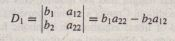This expression is identical to the numerator of the solution (6.3-3). Thus the solution can be expressed as the ratio of the two determinants x = D1/ D. Next form the determinant D2 by replacing the second column of D with the
coefficients on the right side of the equation set. Thus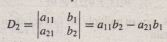This expression is identical to the numerator of the solution (6,3-4). Thus
y = D 2 / D.
Cramer’s method expresses the solutions as ratios of determinants, and thus . can be extended to equations with more than two variables by using determinants having the appropriate dimension. Before using Cramer’s method, be sure variables are lined up in a consistent order (for example, x, y, z) in each equation and move all constants to the right side. Equations (6.1-1) through (6.1-3) fro Example 6.1-1 illustrate this process.
-x + y +2z = 2
3x – y +z = 6
-x +3y+4z =

Cramer’s determinant for this set is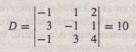One advantage of Cramer’s method is that you can find only one of the unknowns directly if that is all you want. For example, the first unknown is found from x = DJ/ D, where D) is the determinant formed by replacing the
first column in the determinant D with the coefficients on the right side of the equation set: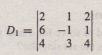This determinant has the value D) ~ 10, and thus x = DJ/ D = 10/10 = 1.
Similarly, y = D 2/ D := -10/10 = -1 and z = D 3 / D = 20/10 = 2, where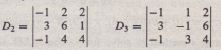Cramer’s Determinant and Singular Problems
When the number of variables equals the number of equations, a singular problem can be identified by computing Cramer’s determinant D. If the determinant is zero, the equations are singular because D appears in the denominator of the solutions. For example, for the set
3x – 4y = 5
6x – 8y = 3
Cramer’s determinant is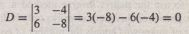Thus the equation set is singular.
Another example is given by the following homogeneous set:
6x +ay = 0
2x +4y = 0
We saw earlier that any finite values of x and y, such that x =-2y, are solutions of this set if a = 12.If a = 12,the only solution is x = y = 0.Cramer’s determinant is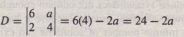and D = 0 if a = 12.Thus the set is singular if a = 12.

In general, for a set of homogeneous linear algebraic equations that contains the same number of equations as unknowns, a nonzero solution exists only if the set is singular; that is, if Cramer’s determinant is zero; furthermore, the solution is not unique. If Cramer’s determinant is not zero, the homogeneous set has a zero solution; that is, all the unknowns are zero. Cramer’s determinant gives some insight into ill-conditioned problems, which are close to being singular. A Cramer’s determinant close to zero indicates an ill-conditioned problem.